# Unit 2 Lesson 2 Scientific Tools and Measurement

• Slides: 20Unit 2 Lesson 2 Scientific Tools and MeasurementUnit 2 Lesson 2 Scientific Tools and Measurement For Good Measure What is measurement? • The ability to describe an observation is important. • A description is a statement that reports what has been observed. • A measurement is a description that includes a number and a unit. Copyright © Houghton Mifflin Harcourt Publishing CompanyUnit 2 Lesson 2 Scientific Tools and Measurement Why do we use standard units of measurement? • Measurements were once based on body parts, such as arms and feet. • This was not accurate because body parts vary in size from person to person. • Using standard units makes it possible for a person in one place to work with the same quantity as someone kilometers away. Copyright © Houghton Mifflin Harcourt Publishing Company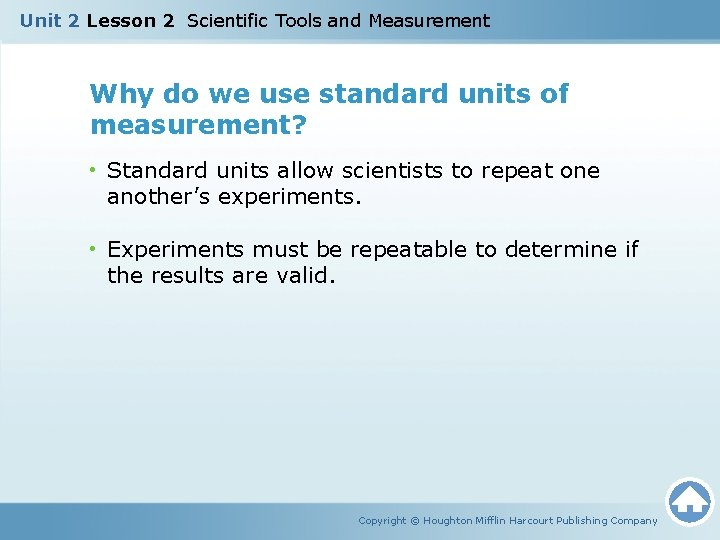Unit 2 Lesson 2 Scientific Tools and Measurement Why do we use standard units of measurement? • Standard units allow scientists to repeat one another’s experiments. • Experiments must be repeatable to determine if the results are valid. Copyright © Houghton Mifflin Harcourt Publishing CompanyUnit 2 Lesson 2 Scientific Tools and Measurement What is the International System of Units? • The modern metric system is called the International System of Units (SI). • The SI units are the language for all scientific measurements. • There are seven base units used to express the different quantities: length, mass, time, temperature, amount of substance, electric current, and light intensity. Copyright © Houghton Mifflin Harcourt Publishing CompanyUnit 2 Lesson 2 Scientific Tools and Measurement What are the advantages of using the SI? • SI measurements provide a common international language for scientists to use to share and compare observations and results. • Changing from one unit to another is easier in SI than other systems because most SI units are based on the number 10. • Conversions in non-SI systems, such as converting from feet to miles, are more complicated. Copyright © Houghton Mifflin Harcourt Publishing Company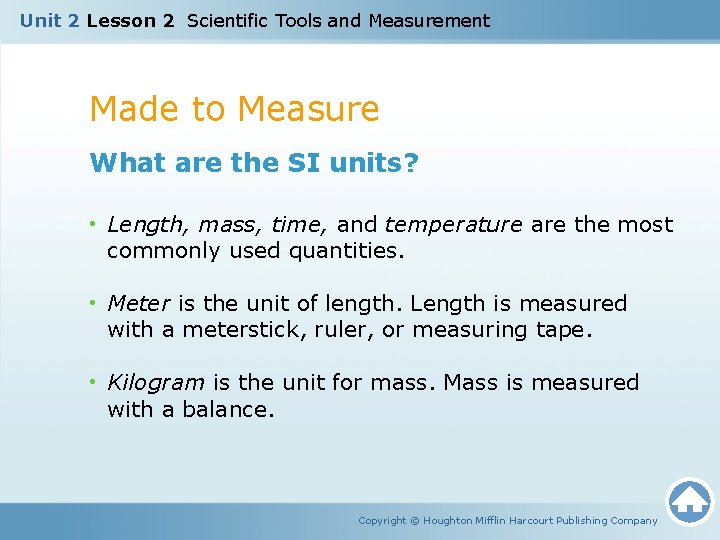Unit 2 Lesson 2 Scientific Tools and Measurement Made to Measure What are the SI units? • Length, mass, time, and temperature are the most commonly used quantities. • Meter is the unit of length. Length is measured with a meterstick, ruler, or measuring tape. • Kilogram is the unit for mass. Mass is measured with a balance. Copyright © Houghton Mifflin Harcourt Publishing Company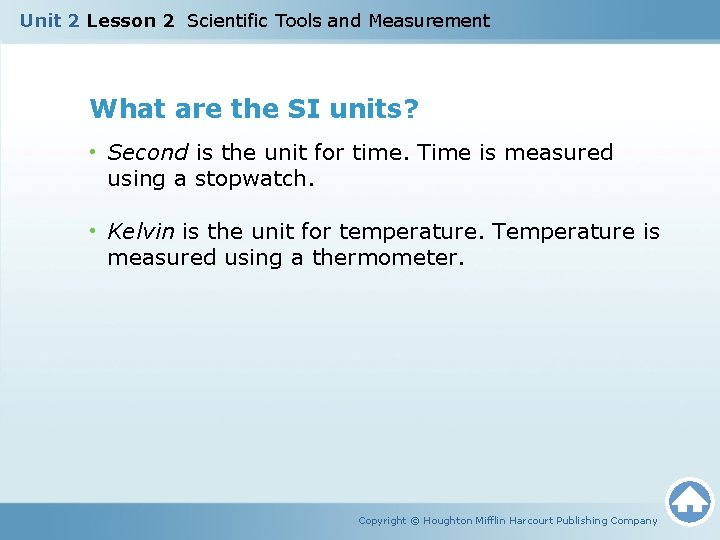Unit 2 Lesson 2 Scientific Tools and Measurement What are the SI units? • Second is the unit for time. Time is measured using a stopwatch. • Kelvin is the unit for temperature. Temperature is measured using a thermometer. Copyright © Houghton Mifflin Harcourt Publishing CompanyUnit 2 Lesson 2 Scientific Tools and Measurement What are the SI units? • Some units are derived units. A derived unit is calculated from a base unit. • Volume is the amount of space something occupies. The SI unit for volume is the cubic meter (m 3). Volume is measured using graduated cylinders and beakers. • Liquid volume is expressed in liters (L), which is not an SI unit. One milliliter (ml) is equal to one cubic centimeter (cm 3). Copyright © Houghton Mifflin Harcourt Publishing CompanyUnit 2 Lesson 2 Scientific Tools and Measurement What are the SI units? • Weight is the measurement of the gravitational force on an object. The SI unit for weight is the newton (N). • Weight is measured using a spring scale and depends on the object’s mass. • Measurements such as density must be calculated. Density is calculated by dividing an object’s mass by its volume. Copyright © Houghton Mifflin Harcourt Publishing CompanyUnit 2 Lesson 2 Scientific Tools and Measurement How can we make very large or small measurements easy to work with? • A prefix is one or more letters or syllables added to the beginning of a word to change its meaning. • SI uses prefixes to express an SI unit that is larger or smaller than the base unit. • Kilo- means 1, 000 times, so a kilogram is 1, 000 grams. • Milli- indicates 1/1, 000 times, so a millimeter is 1/1, 000 of a meter. Copyright © Houghton Mifflin Harcourt Publishing CompanyUnit 2 Lesson 2 Scientific Tools and Measurement How can we make very large or small measurements easy to work with? • Scientific notation is a short way of representing very large numbers or very small numbers. • Numbers in scientific notation are written in the form a × 10 b. • The value of a is usually a number between 1 and 10. • Locate the decimal point and move it to the left or right until it is immediately before or after the numeral that becomes a. Copyright © Houghton Mifflin Harcourt Publishing Company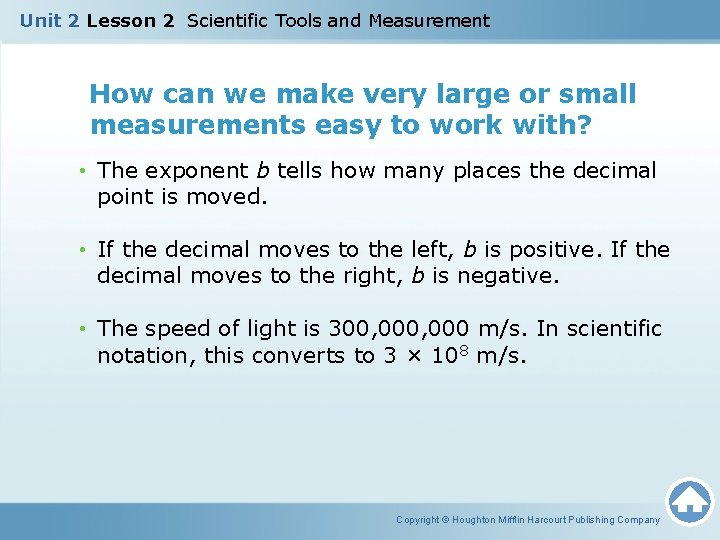Unit 2 Lesson 2 Scientific Tools and Measurement How can we make very large or small measurements easy to work with? • The exponent b tells how many places the decimal point is moved. • If the decimal moves to the left, b is positive. If the decimal moves to the right, b is negative. • The speed of light is 300, 000 m/s. In scientific notation, this converts to 3 × 108 m/s. Copyright © Houghton Mifflin Harcourt Publishing Company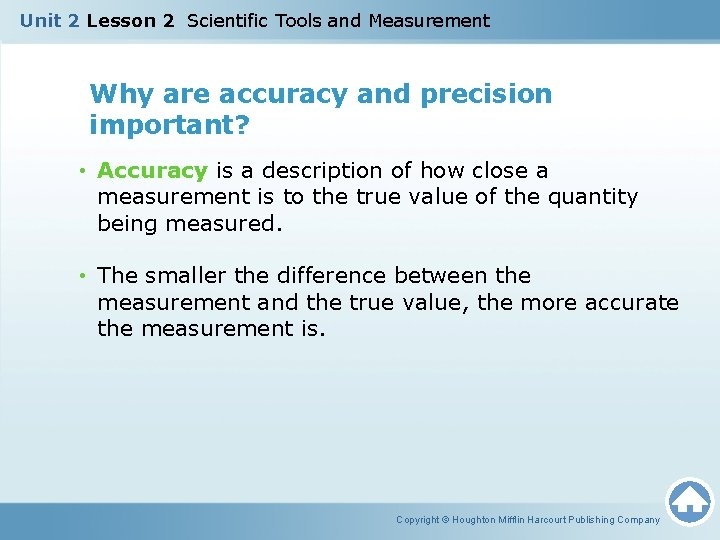Unit 2 Lesson 2 Scientific Tools and Measurement Why are accuracy and precision important? • Accuracy is a description of how close a measurement is to the true value of the quantity being measured. • The smaller the difference between the measurement and the true value, the more accurate the measurement is. Copyright © Houghton Mifflin Harcourt Publishing Company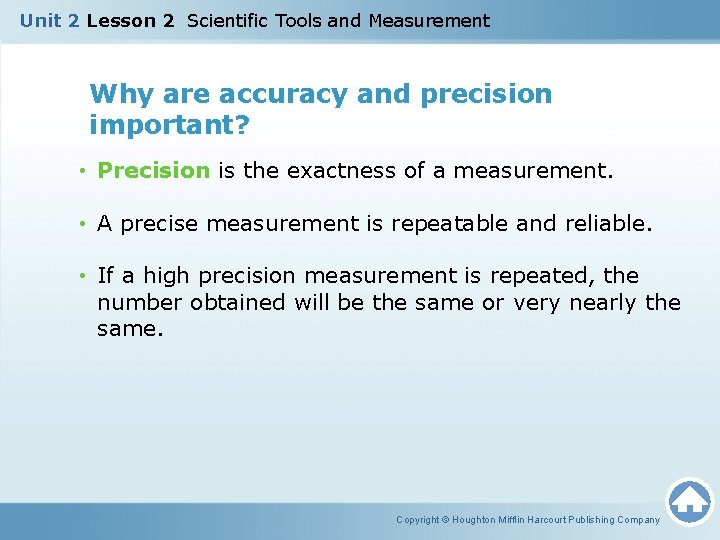Unit 2 Lesson 2 Scientific Tools and Measurement Why are accuracy and precision important? • Precision is the exactness of a measurement. • A precise measurement is repeatable and reliable. • If a high precision measurement is repeated, the number obtained will be the same or very nearly the same. Copyright © Houghton Mifflin Harcourt Publishing CompanyUnit 2 Lesson 2 Scientific Tools and Measurement Why are accuracy and precision important? • How do these diagrams represent accuracy and precision? Copyright © Houghton Mifflin Harcourt Publishing Company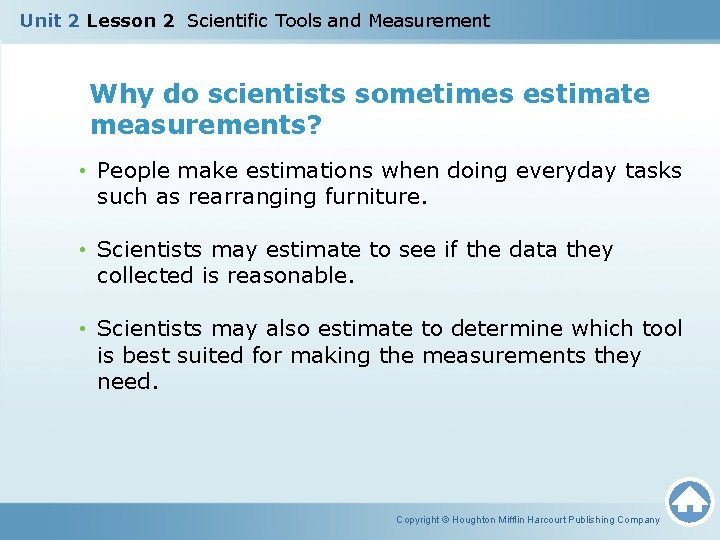Unit 2 Lesson 2 Scientific Tools and Measurement Why do scientists sometimes estimate measurements? • People make estimations when doing everyday tasks such as rearranging furniture. • Scientists may estimate to see if the data they collected is reasonable. • Scientists may also estimate to determine which tool is best suited for making the measurements they need. Copyright © Houghton Mifflin Harcourt Publishing Company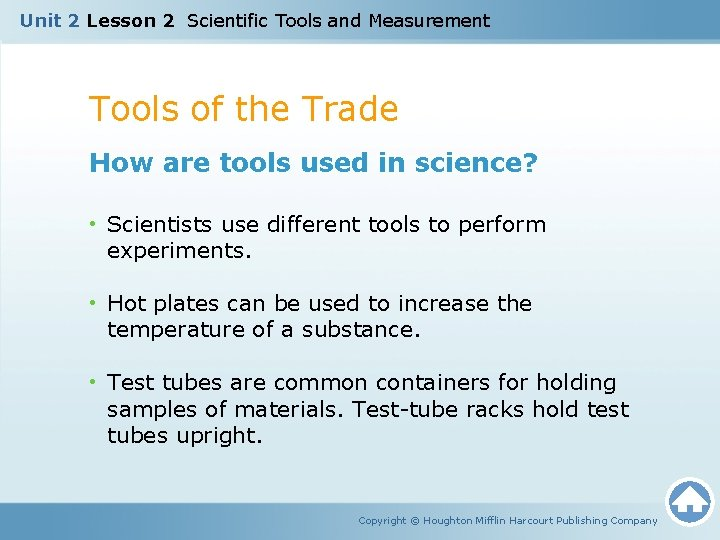Unit 2 Lesson 2 Scientific Tools and Measurement Tools of the Trade How are tools used in science? • Scientists use different tools to perform experiments. • Hot plates can be used to increase the temperature of a substance. • Test tubes are common containers for holding samples of materials. Test-tube racks hold test tubes upright. Copyright © Houghton Mifflin Harcourt Publishing Company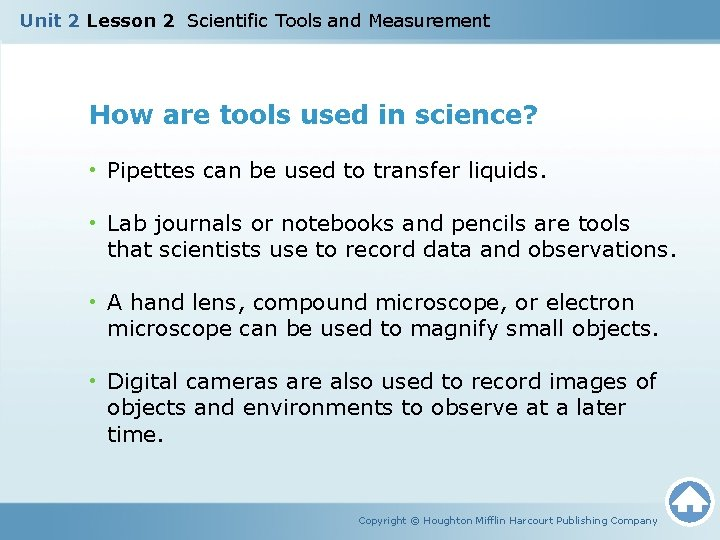Unit 2 Lesson 2 Scientific Tools and Measurement How are tools used in science? • Pipettes can be used to transfer liquids. • Lab journals or notebooks and pencils are tools that scientists use to record data and observations. • A hand lens, compound microscope, or electron microscope can be used to magnify small objects. • Digital cameras are also used to record images of objects and environments to observe at a later time. Copyright © Houghton Mifflin Harcourt Publishing CompanyUnit 2 Lesson 2 Scientific Tools and Measurement How are computers and technology used by scientists? • The use of science for practical purposes is called technology. New technology leads to new discoveries. • Calculators and computers allow scientists to compute, display, analyze, record, and model data. They even help scientists to publish their findings. • Probeware, which is a measuring tool linked to a computer, can be used to obtain and display the values of a quantity for long-term study. Copyright © Houghton Mifflin Harcourt Publishing Company